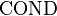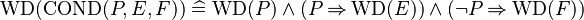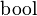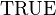# Extended Operator Translation

## Purpose

Most external provers support only the core mathematical language without any mathematical extension. Consequently, when calling such a prover, any predicate (hypothesis or goal) which contains some mathematical extension is ignored. This is quite annoying, especially when the extension does not play any role in the proof, but is just in the way of the prover.

Since Rodin 2.8, there is support in the AST library and the Sequent prover for translating datatypes into the plain mathematical language. This allows to use external provers on sequents that contain datatypes.

The purpose of this development is to provide a similar mechanism for the mathematical operators.

## Specification

We first recall the way extended operators are defined and the associated semantics, then we define a translation scheme from operators to plain set theory.

### Operator Syntax

An extended operator is a mathematical extension which is introduced using the Theory plug-in. It is specified by giving a list of arguments, which can be expressions or predicates, and a return kind (here again, predicate or expression). The list of argument is either fixed or of unbounded length (e.g., several expressions).

Operators can be generic (i.e., polymorphic), in which case they also take implicit type parameters.

### Operator Semantics

In most cases, the semantics of operators is expressed by defining an equivalent formula which represents the operator applied to its formal arguments.

Alternatively, an operator can be defined axiomatically by specifying its properties.

In all cases, an operator can be partial, in which case a well-definedness condition needs to be proven for each occurrence of the operator.

### Translation Scheme

The purpose of the translation is to replace an occurrence of an operator by a function application. As operators are polymorphic, several occurrences of the same operator might be replaced by several function applications.

#### Well-Definedness

A function application is WD-strict: the well-definedness of a function application implies the well-definedness of each of its arguments. Consequently, this translation can only be applied to WD-strict operators. Indeed, if you consider the operator$\mathrm{COND}$, its well-definedness condition is$\mathrm{WD}(\mathrm{COND}(P, E, F))\defi \mathrm{WD}(P)\land (P\limp \mathrm{WD}(E)) \land (\lnot P\limp \mathrm{WD}(F))$ .

Therefore, there is no possibility to replace this operator with a function application.

#### Function Creation

An extended operator can take a mix of expressions and predicates as argument, and can return either a predicate or an expression. To replace it with a function application, we thus need to translate in both directions from predicates to expressions. This is easily done by using the$\bool$ operator in one direction and equality with$\True$ in the other direction.

To resolve the polymorphism of extended operators, we need to distinguish operator occurrences by their signature, that is a tuple composed of the types of their expression arguments, the number of their predicate arguments and the nature of their result (either predicate, or expression with a specific type). The translation should generate one fresh function identifier for each signature.

#### Soundness of the Translation

As concerns the partiality of the extended operator, the exact domain of the operator is only available to the Theory plug-in. The AST library has no access to it. The idea of the translation is to replace each occurrence of a mathematical operator by a total function. Replacing a partial operator by a total function is sound, because the sequent to prove is known to be well-defined. This guarantees that partial operators are never used outside of their domains, and thus can soundly be extended arbitrarily in order to become total.

Consequently, this translation scheme does not introduce any soundness issue. On the other hand, it is not complete as part of the extended operator specification has been lost in translation.